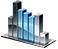Object Account

# Object Account - account status

### Account Properties

• int Leverage - account leverage
• double Balance - current account balance
• double MarginLocked - current margin requirements
• double Equity - balance including open positions (equity)
• double MarginFree - free margin
• int MarginLevel - margin level
• double AvailablePos - total available position
• int AccountID - ID of the current account
• int MarginShort - short margin level
• string Valute - deposit currency

Example:

` int Initialize() {return(0);} int Run() { System.Print("Leverage = "+Account.Leverage); System.Print("Balance = "+Account.Balance); System.Print("MarginLocked = "+Account.MarginLocked); return(0); } int DeInitialize(){return(0);} `